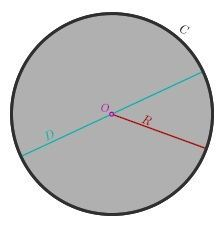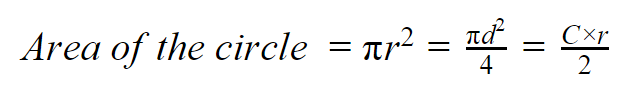# Area of a Circle Formula

A circle is a round figure that consists of points equidistant from a certain or fixed point from a central point of the circle. A central point from where the radius is drawn is known as the center point of a circle. The radius of a circle is the distance from the center of a circle to any point on circle. If you place two radii end-to-end in a circle, you would have the same length as one diameter. Thus, the diameter of a circle is twice as long as a radius.  The area of circle is the number of square units inside that circle. A circle has a maximum possible area for a given perimeter and the minimum possible perimeter for a given area. The perimeter C of circle is called the circumference.The Area of a Circle Formula is given as,Where
r is the radius of the circle.
d is the diameter of the circle.
C is the circumference of the circle.

### Solved Examples

Question 1: Find the area of the circle whose radius is 8 cm?
Solution:

Given,
Radius of a circle = r = 8 cm
Area of a circle
π r2
π × 82 cm2
π × 64 cm2
= 200.96 cm2